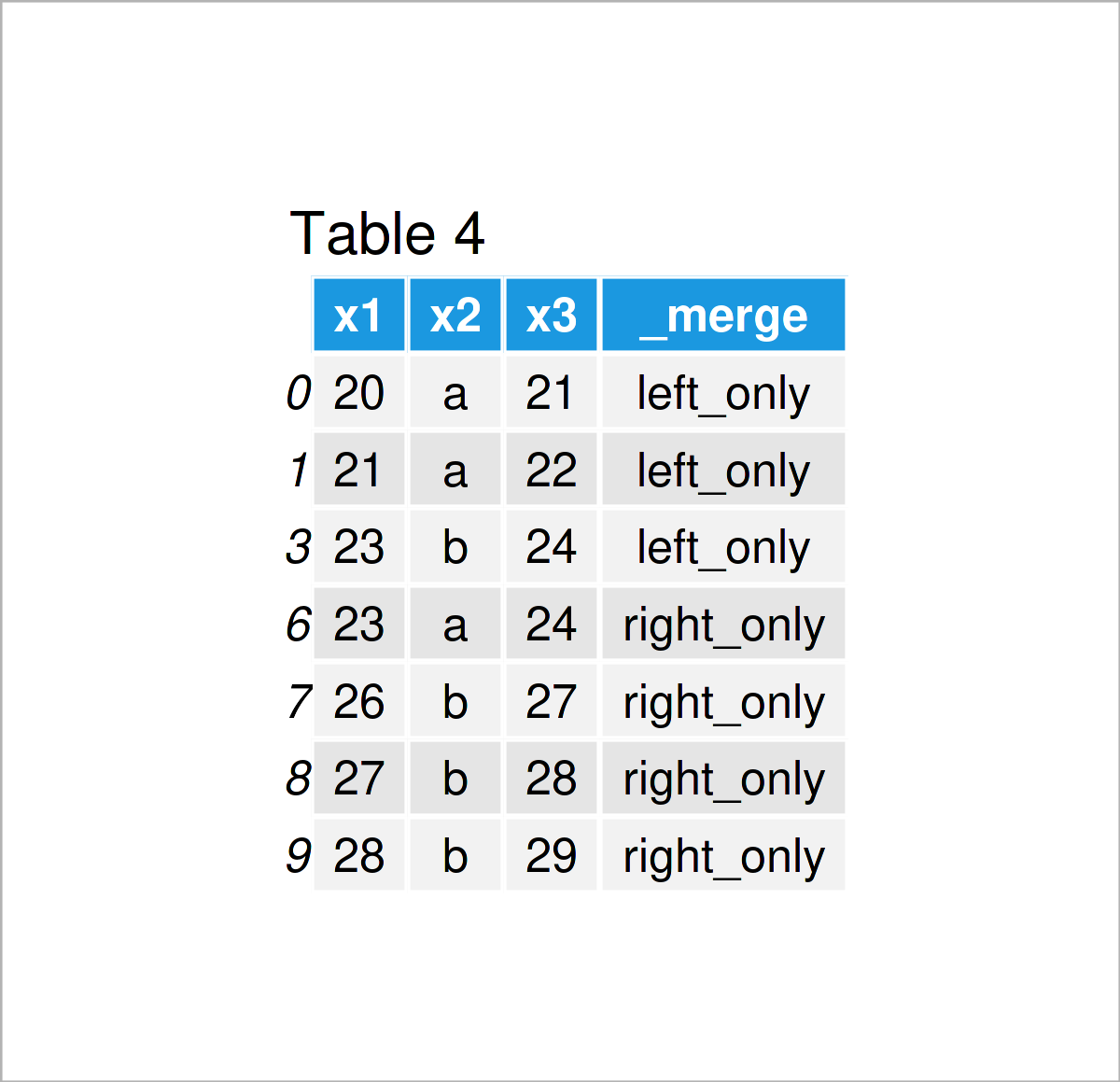# Compare Two pandas DataFrames in Python (Example) | Find Differences Row by Row

In this tutorial, I’ll show how to find different rows between two pandas DataFrames in the Python programming language.

The article looks as follows:

So without further additions, let’s do this…

## Example Data & Add-On Libraries

First, we need to import the pandas library:

`import pandas as pd                                    # Import pandas library in Python`

As a next step, I also have to create two exemplifying pandas DataFrames:

```data1 = pd.DataFrame({'x1':range(20, 26),              # Create first pandas DataFrame
'x2':['a', 'a', 'a', 'b', 'b', 'b'],
'x3':range(21, 27)})
print(data1)                                           # Print first pandas DataFrame``````data2 = pd.DataFrame({'x1':range(22, 29),              # Create second pandas DataFrame
'x2':['a', 'a', 'b', 'b', 'b', 'b', 'b'],
'x3':range(23, 30)})
print(data2)                                           # Print second pandas DataFrame```After executing the previous Python programming code the two pandas DataFrames visualized in Tables 1 and 2 have been created.

As you can see, both data sets contain the same column names, but partly different rows.

## Example: Find Different Rows in Two pandas DataFrames Using merge() Function

In this example, I’ll show how to compare two pandas DataFrames with different lengths and identify all different rows that are contained in only one of the two DataFrames.

As a first step, we have to apply the merge function to append both of our DataFrames vertically.

Note that we are using an outer join, and we are setting the indicator argument to be equal to True so that we can identify later which of the rows are contained in both data sets:

```data_12 = data1.merge(data2,                           # Merge DataFrames with indicator
indicator = True,
how = 'outer')
print(data_12)                                         # Print merged DataFrames```As shown in Table 3, the previous Python syntax has created a new pandas DataFrame that combines the values in our two input data sets. Furthermore, we have added a new column that indicates whether a row was contained in both or only one of the data sets.

If we want to return only the different rows, we can use the loc attribute of our concatenated DataFrame as shown below:

```data_12_diff = data_12.loc[lambda x : x['_merge'] != 'both']  # Remove shared rows
print(data_12_diff)                                    # Print differences between DataFrames```In Table 4 you can see that we have managed to create another pandas DataFrame with the previously shown Python code. This DataFrame contains only the differences between our two input data sets as well as an indicator showing which of the input data sets has contained the corresponding row.

## Video, Further Resources & Summary

In case you need more explanations on how to match the rows of multiple pandas DataFrames, I recommend watching the following video that I have published on my YouTube channel. I’m explaining the Python code of this article in the video:

In addition, you could read the related articles which I have published on this website. You can find a selection of tutorials on topics such as extracting data and merging below.

At this point you should have learned how to find intersections and return only different rows between two pandas DataFrames with different lengths in Python. Tell me about it in the comments section, if you have further questions.

Subscribe to the Statistics Globe Newsletter

•Leandro
November 27, 2022 10:23 pm

Hello! Thank you so much for this tutorial. I was getting crazy that already.

•November 30, 2022 10:38 am